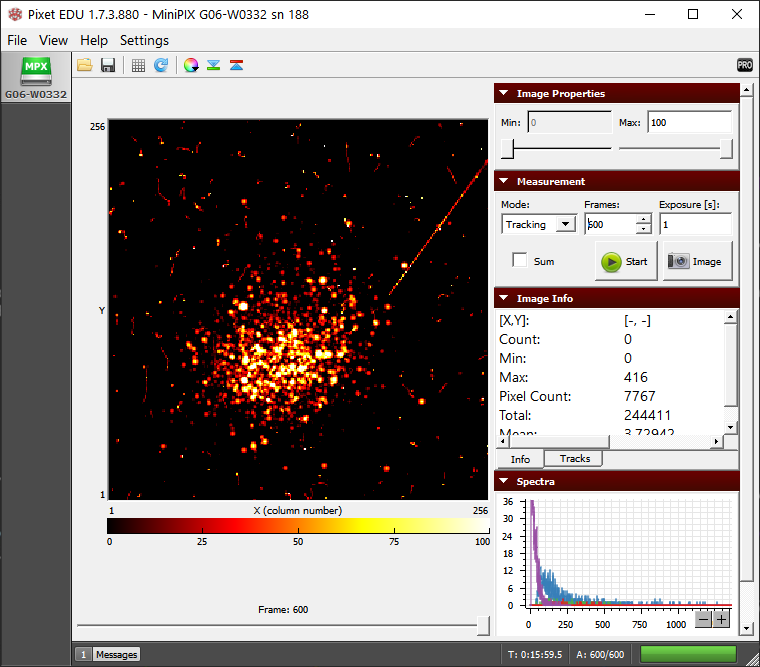# Estimating the Thickness of Aluminum Foil

## Equipment

Stage, camera holder, source holder, polonium-210, aluminum foil (Reynolds wrap standard) , Minipix-EDU

## Procedure

1. Launch the Pixet Basic software and modify settings to the following:
1. Min Level: 0
2. Max Level: 100
3. Measurement Mode: Tracking
4. Frames: 600
5. Exposure: 1 s
6. Sum: check
7. Color Map: Hot
2. Mount the MiniPix EDU camera and polonium wrapped in a single layer of aluminum foil on the stage, as shown in figure 1.
Note: Using a heavy-duty foil might alter the results.Figure 1. The setup of polonium source wrapped in aluminum foil

3. Keep the source and camera as close as possible and click on the play button.
4. Next, wrap two foils of aluminum and without moving the stage, collect the data again.

## Results

1. Initially, we observe some traces of alpha particle blobs (Fig. 2). The muon (a long streak) observed is not because of aluminum foil but is a rare observation which we may have in any experiment we perform. Basically, it is part of background radiation.Figure 2. Alpha particles passing through a single layer of aluminum foil

2. The blobs of alpha particles disappear when two layers of foil are used.
3. The mean linear range (R) of alpha particles in the air can be calculated using the following equation:where Ek is the kinetic energy of alpha particles in MeV. From the previous experiments, we know that the energy of alpha particles is approximately 4.2 MeV when the source and cameras are placed as close as possible. Thus, considering the Ek to be 4.2 MeV, we will calculate the mean linear range of alpha particles in aluminum (Z=13) using the following formula:where Z is the atomic number and ⍴ is the density of the substance blocking material (⍴Al = 2700 kg/m3).

## Conclusion

1. Since the alpha particle was able to go through a single layer of foil, that means the foil is thinner than 13.02 µm (Fun fact: Human hair diameter is ≈ 70 µm).
2. But, two layers of foil were able to block the alpha particles, which means that the foil is thicker than 6.5 µm. Thus we can conclude that the thickness is within the range of 6.5 µm to 13.02 µm.